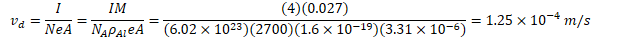# Electric Current

• Electric current is the flow rate of electric charge

• Units are C/s, or amperes (A)

• Positive current flow is the direction of the flow of positive charges, which is opposite the direction of electron flow

•# Drift Velocity

• In a conductor, electrons are inconstant thermal motion.

• Net electron flow, however, is zero because the motion is random

• When an electric field is applied, a small net flow in a direction opposite the electric field is observed

•# Derivation of Current Flow# Current Density

•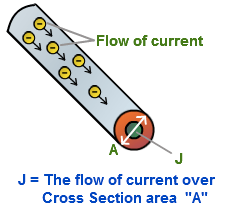# Resistance

• Resistance is the ratio of the potential drop across an object to the current flowing through the object.

• Object which have a fixed resistance (not a function of current of potential drop) are known as Ohmic materials and are said to follow Ohm's Law (an empirical law)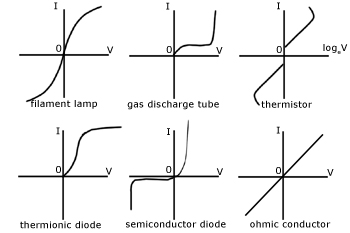# Resistance of a Wire

• The resistance of a wire depends on the geometry of the wire as well as a material property known as resistivity ⍴ (Unit: Ω·m)

• Resistivity relates to the ability of a material to resist the flow of electrons# Refining Ohm's Law

••••# Conversion of Electric Energy to Thermal Energy

•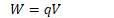••# Example 1: Silver Wire

•1. Determine the resistance of the wire

•2. Determine the current flowing through the wire

•3. Determine the drift velocity of the free electrons in the wire (assume one free electron per atom)

••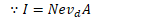•4. Determine the average time required for electrons to pass from the negative terminal of the battery to the positive terminal

•# Example 2: Aluminum Wire

•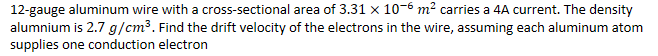•